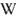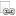back to index

# Method - reuse of VU meters

## Problem

A VU meteris a cheap, relatively abundant kind of an electromechanic measuring instrument. There are many available on the market in a variety of sizes, shapes, and often low prices, sometimes even salvageable for free.

A VU meter can serve as a voltmeteror ammeter. Its accuracy is often low and the coil parameters aren't optimal for either voltmeter or ammeter, however the cost advantage makes it a tempting solution in many low-end applications.

## Meter characteristics

The VU meter is a moving-coil galvanometer. Electrically it is characterized mainly by the resistance of its coil and the current needed for full deflection.

An example combination is 650Ω/200µA. The corresponding full-deflection voltage on the coil, according to the Ohm's law, is therefore 130 millivolts.

The disadvantage for some applications and for calibration is the nonlinearity of VU meters; they tend to be more responsive in the left part of the scale. Another disadvantage, though that may be shared with other kinds of meters in a given application, is the need for calibration of the dial; dB or percentages of signal strength are rarely suitable.

The described methods can be used for any galvanometer-based meter. An unknown panel meter can be stripped from its series resistor or a shunt, measured, new resistor added, and its dial redrawn.

## MeasurementsAn unknown VU meter (and any galvanometer-based meter in general) can be measured using a source of suitably low voltage and a suitably-sized variable serial resistor. 3.3 or 5V and 100 to 220 kiloohm pot are usually sufficient.

Two of the full-deflection current, full-deflection voltage, and coil resistance have to be measured. The described method uses direct simultaneous measurement of current and voltage; both can be measured one at a time if only one multimeter is available or if extra high precision is desired (the megaohm-range of the voltmeter impedance can however be usually neglected).

The circuit is set, the pot is set to maximum resistance, and the power is applied. The pot is gradually turned down, increasing current through the circuit until the meter's needle deflects to the desired value (usually the end of the scale).

For this example, the Small Power Supplywas used, set to 3.3V output. This power supply is also a real-life example of use of VU meters for both a voltmeter and an ammeter.Measuring circuitMeasuring circuit detailMeasuring circuit detailMeasuring circuit, detail of connections to power supply

## Uses### Voltmeter

For a voltmeter, the meter is used to measure current through the circuit. The current is limited with a serial resistor. Its value is calculated from the full-deflection current and the desired full-deflection input voltage.

Let's assume a 200µA full-deflection current and desire to measure 15 volts. The resistance to achieve 200µA from 15V is (R=U/I, R=15/0.0002=75000) 75 kΩ. This value includes the resistance of the meter's coil, however in this case it is lower than 1% of the total and can be safely neglected as it is below the accuracy of the meter.

Correspondingly, for a serial resistor of 72 kΩ the full-range voltage is 14.4V and for 81 kΩ it is 16.2V.

### Ammeter

For an ammeter, the meter is used to measure voltage over a shunt resistor. The resistor has to develop needed voltage at maximal measured current to provide enough millivolts for the meter connected in parallel. Its value is therefore calculated from the full-deflection voltage of the meter and the full-range current.

Let's assume the same 200µA meter, with 650Ω coil and corresponding 130mV full deflection. For measuring a 2-ampere current, the shunt value is (R=U/I, R=0.13/2=0.065) 0.065 ohm. The power loss on the shunt is (P=U*I, P=0.13*2=0.26) 0.26 watt.

For a 100mA meter, the resistor will be (R=0.13/0.1=1.3) 1.3Ω, and the power loss 0.13W.

For a 10A meter the resistor will be (R=0.13/10=0.013) 0.013 ohm, the power loss will be 1.3W.

The higher the full-deflection voltage on the meter, the higher the resistor value for the given current and the higher the power loss and corresponding shunt heating. The higher is also the voltage loss and that has to be taken in account.

Higher-value shunts are best achieved by using off-the-shelf resistors with sufficient wattage. Lower-value ones, in fraction-of-ohm area, are best made from a suitable piece of resistive wire with sufficient thickness and low temperature coefficient.

### Use with AC voltage

The meters as described are suitable for use with DC voltage. For AC, the current has to be rectified, usually using a [link?:diode bridge]. The meter has to be calibrated with AC, as if calibrated with DC it will show the average value instead of the RMS. This assumes constant waveform. For unpredictable one, a True RMS converterhas to be employed.

For measuring higher AC currents, a current transformercan be used.

## TODO

• Description of calibration and dial drawing
• Description of adding a backlight
• Javascript calculator for the resistors
• Current transformer use

## ImagesMeter AMeter BMeter C